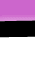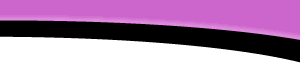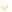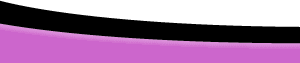HomeFAQsTutorialsResourcesHelpDreamweaverFAQ.com » Tutorials » JavaScript » Forms And Math Page 4# Forms and Math page 4

## Form Calculations and Special Discounts

A discount may be in many formats, but a percentage of the sales price above a certain figure is the most common. We'll create a stand-alone function that will calculate the discount, a "generic" function that can be used again and again. The function will contain 3 arguments, val, threshold and rate, and it will return the variable discount. We'll express the rate argument as a percentage.
For example: calculateDiscount(this.form.sub.value,150,"5%") Try it!

`<script language="JavaScript"><!--function calculateDiscount(val,threshold,rate){  var discount=(val>threshold)? (val-threshold)*parseFloat(rate)/100:0;  return discount;} // --></script>`

The above concept is developed further to create this form:

Enter # of widgets @\$29.90 each

Price (Remember this value is not used in the calculation. The Price is stored in a hidden field.)

Subtotal(widgets x price)

5% discount on orders over \$150.00

Total (Subtotal - discount)

The final code for the form above would look like this:
```<script language="JavaScript"><!--function calculateDiscount(val,threshold,rate){  var discount=(val>threshold)? (val-threshold)*parseFloat(rate)/100:0;  return discount;}

function calculate(){  with(document.maths){	  if(widgets.value<=0){		  alert("Positive numbers only, please"); return;	  }	  if (widgets.value % 1!=0){		  if(window.confirm("Round up to "+Math.ceil(widgets.value)+"?"))
{			  widgets.value=Math.ceil(widgets.value);			  }		  else{			  return;		  }	  }	  var Sub=widgets.value*hiddenField.value;	  var Disc=calculateDiscount(Sub,150,5);	  discount.value= Disc? to_currency(Disc,"\$"):0;	  subtotal.value =to_currency(Sub,"\$");	  total.value= to_currency(Sub-Disc,"\$");  }}// --></script>
```

`<form name="maths"><p><input type="text" name="widgets" size="8">Enter # of widgets @\$29.90 each </p><p><input type="text" name="price" value="\$29.90" size="8">Price</p><p><input type="text" name="subtotal"	size="8">Subtotal(widgets x price)</p><p><input type="text" name="discount" size="8">5% discount on orders over \$150.00</p><p><input type="text" name="total" value="Total" size="10">Total(Subtotal - discount)</p><p><input type="button" name="Button" value="Calculate" onClick="calculate()"></form`Copyright © 2001-2021 DreamweaverFAQ.com All Rights Reserved.